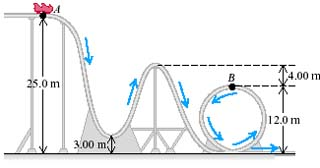# Problem: A 350 kg roller coaster starts from rest at point exttip{A}{A} and slides down the frictionless loop-the-loop shown in the accompanying figure.A. How fast is this roller coaster moving at point B exttip{B}{B}?B. How hard does it press against the track at point Bexttip{B}{B}?

###### FREE Expert Solution

We'll use the law of conservation of energy:

$\overline{){{\mathbf{K}}}_{{\mathbf{i}}}{\mathbf{+}}{{\mathbf{U}}}_{{\mathbf{i}}}{\mathbf{+}}{{\mathbf{W}}}_{{\mathbf{nc}}}{\mathbf{=}}{{\mathbf{K}}}_{{\mathbf{f}}}{\mathbf{+}}{{\mathbf{U}}}_{{\mathbf{f}}}}$, where Wnc is the work done by non-conservative forces such as friction.

Potential energy:

$\overline{){\mathbf{U}}{\mathbf{=}}{\mathbf{m}}{\mathbf{g}}{\mathbf{h}}}$

Kinetic energy:

$\overline{){\mathbf{K}}{\mathbf{=}}\frac{\mathbf{1}}{\mathbf{2}}{{\mathbf{mv}}}^{{\mathbf{2}}}}$

A.

In this problem, we don't have no-conservative forces, and hence Wnc = 0.

95% (289 ratings)###### Problem Details

A 350 kg roller coaster starts from rest at point and slides down the frictionless loop-the-loop shown in the accompanying figure.A. How fast is this roller coaster moving at point B ?

B. How hard does it press against the track at point B?

Frequently Asked Questions

What scientific concept do you need to know in order to solve this problem?

Our tutors have indicated that to solve this problem you will need to apply the Motion Along Curved Paths concept. You can view video lessons to learn Motion Along Curved Paths. Or if you need more Motion Along Curved Paths practice, you can also practice Motion Along Curved Paths practice problems.

What professor is this problem relevant for?

Based on our data, we think this problem is relevant for Professor Chapagain's class at FIU.